## Circular Polarization Condition of 2 Linearly Polarized Waves

### [Problem]

Show the condition such that the total field of two linearly polarized waves with crossing angle 꺠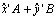becomes a circularly polarized wave, where A and B are complex numbers.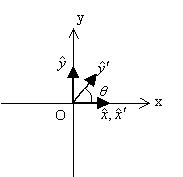### [Animation]

Example 1 (Circularly polarized wave)

An animation of the total field of A=ra exp(j 꺠a) and B=rb exp(j 꺠b) in the case that 꺠=30걢,ra=rb=1,꺠a=0걢,꺠b=꺠+180걢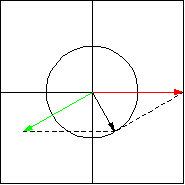Red겏 : x'A (linear polarization along x' axis)
Green겏 : y'B (linear polarization along y' axis)
Black겏 : x'A+y'B (total field, circularly polarization)

Example 2 (Circularly polarized wave)

An animation of the total field of A=ra exp(j 꺠a) and B=rb exp(j 꺠b) in the case that 꺠=90걢,ra=rb=1,꺠a=0걢,꺠b=꺠+180걢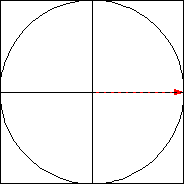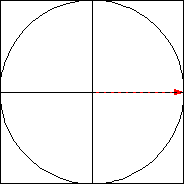Example 3 (Elliptically polarized wave)

An animation of the total field of A=ra exp(j 꺠a) and B=rb exp(j 꺠b) in the case that 꺠=90,ra=1,rb=1/2,꺠a=0걢,꺠b=꺠+180걢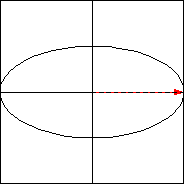Go Back